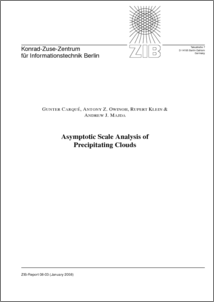Repository: Freie Universität Berlin, Math Department

# Asymptotic Scale Analysis of Precipitating Clouds

Carqué, G. and Owinoh, A.Z. and Klein, R. and Majda, A. J. (2008) Asymptotic Scale Analysis of Precipitating Clouds. ZIB-Report, 08-03 . ISSN 1438-0064Preview

273kB

Official URL: http://opus.kobv.de/zib/volltexte/2008/1083/

## Abstract

Asymptotic analyses of the three dimensional compressible flow equations coupled with transport equations for the mixing ratios of water vapour, cloud water and rain water are described. We obtain reduced systems of equations for two particular regimes of length and time scales: Models for the long time evolution of deep convective columns and for the short time evolution of shallow convective layers. The asymptotic deep convective column model is anelastic, yet the vertical motion is pressure free, i.e., it evolves freely in interaction with buoyancy while the horizontal divergence adjusts to fullfil the anelastic constraint. The perturbation pressure guaranteeing compliance with the horizontal divergence constraint obeys a Poisson-type equation. Surprisingly, the vertical velocity plays an important role in the horizontal dynamics through the Coriolis term. The vertical acceleration in a saturated column is directly determined by the buoyancy induced by potential temperature differences relative to the background stratification. This potential temperature deviation is a conserved quantity. Evaporation is the only important microphysical process in the undersaturated regime. The evaporation rate depends on the saturation deficit and the amount of rain water present and determines the (downward) vertical velocity and the distribution of water vapour. To connect the deep convective column solutions to top and bottom boundary conditions, a different flow regime needs to be accounted for. Within shallow layers whose depth is comparable to the column diameters, adjustment to physical boundary conditions can take place. This is the second regime considered in this report. The shallow convective layer regime is shown to be asymptotically described by Boussinesq-type equations. These equations are closed by evolution equations which show that, in the saturated regime, the distributions of potential temperature and cloud water are determined by a condensation rate that is directly proportional to the vertical velocity. In the undersaturated regime, the potential temperature distribution is determined by the amount of rain present, since the water vapour in this case is shown to be a conserved quantity. In both regimes the distribution of rain water depends on the rain water flux.

Item Type: Article atmospheric moist convection , deep convection , shallow convection , precipitating clouds , asymptotic analysis Mathematical and Computer Sciences > Mathematics > Applied Mathematics Department of Mathematics and Computer Science > Institute of Mathematics > Geophysical Fluid Dynamics Group 522 Ulrike Eickers 08 Jul 2009 14:56 03 Mar 2017 14:40

Repository Staff Only: item control page